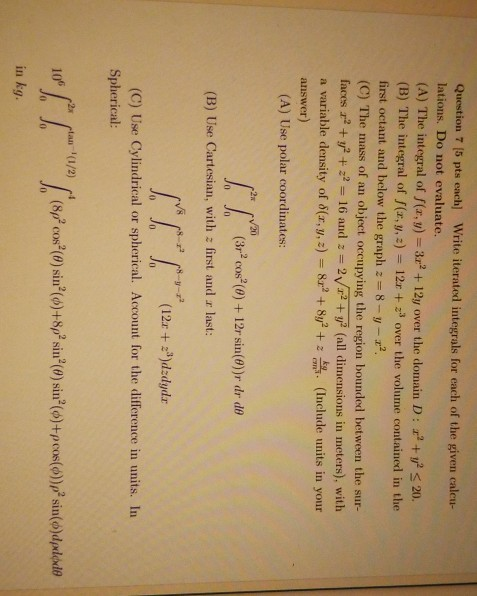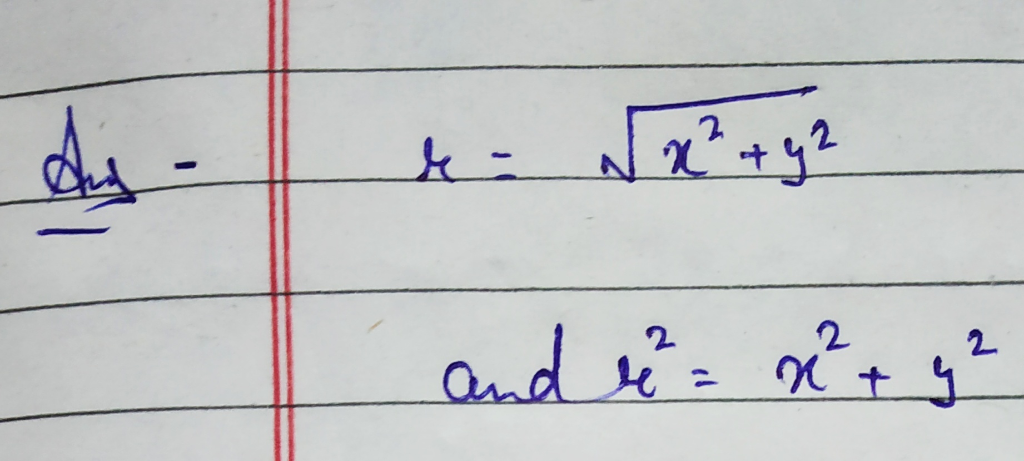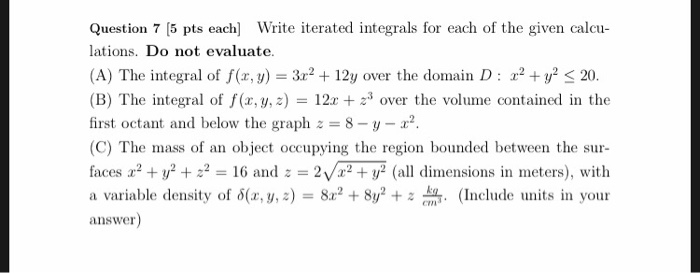Questionsolutions are labeled a to c at the bottom. can you explain what the r stands for. I'm assuming x2 + y2#### Earn Coins

Coins can be redeemed for fabulous gifts.

Similar Homework Help Questions
• ### Question 7 5 pts each Write iterated integrals for each of the given calu lations. Do not evaluate. (A) The integral of f(x, )212y over the domain D: 2 y 20. (B) The integral of f(x, y, z) = 12x...Question 7 5 pts each Write iterated integrals for each of the given calu lations. Do not evaluate. (A) The integral of f(x, )212y over the domain D: 2 y 20. (B) The integral of f(x, y, z) = 12x + 3 over the volume contained in the first octant and below the graph z 8-y 2 (C) The mass of an object occupying the region bounded between the sur faces x2 + y2 + Z2 = 16 and z...